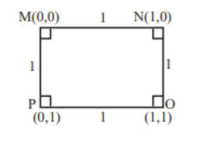# For the four circlesQuestion:

For the four circles $\mathrm{M}, \mathrm{N}, \mathrm{O}$ and $\mathrm{P}$, following four equations are given :

Circle $M: x^{2}+y^{2}=1$

Circle $\mathrm{N}: \mathrm{x}^{2}+\mathrm{y}^{2}-2 \mathrm{x}=0$

Circle $\mathrm{O}: \mathrm{x}^{2}+\mathrm{y}^{2}-2 \mathrm{x}-2 \mathrm{y}+1=0$

Circle $P: x^{2}+y^{2}-2 y=0$

If the centre of circle $M$ is joined with centre of the circle $N$, further centre of circle $\mathrm{N}$ is joined with centre of the circle $\mathrm{O}$, centre of circle $\mathrm{O}$ is joined with the centre of circle $\mathrm{P}$ and lastly, centre of circle $\mathrm{P}$ is joined with centre of circle M, then these lines form the sides of a :

1. (1) Rhombus

2. (2) Square

3. (3) Rectangle

4. (4) Parallelogram

Correct Option: , 2

Solution:

$M: x^{2}+y^{2}=1(0,0)$

$\mathrm{N}: \mathrm{x}^{2}+\mathrm{y}^{2}-2 \mathrm{x}=0(1,0)$

$\mathrm{O}: \mathrm{x}^{2}+\mathrm{y}^{2}-2 \mathrm{x}-2 \mathrm{y}+1=0(1,1)$

$P: x^{2}+y^{2}-2 y=0(0,1)$